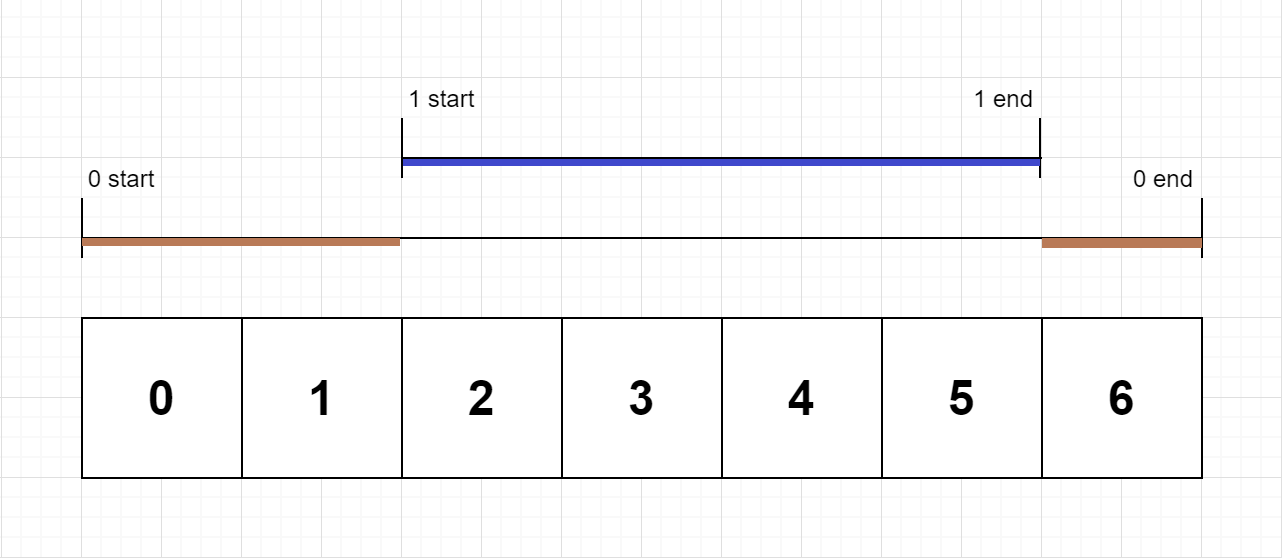# 函数的独占时间

## 636. 函数的独占时间 (Medium)```输入：n = 2, logs = ["0:start:0","1:start:2","1:end:5","0:end:6"]

```

```输入：n = 1, logs = ["0:start:0","0:start:2","0:end:5","0:start:6","0:end:6","0:end:7"]

```

```输入：n = 2, logs = ["0:start:0","0:start:2","0:end:5","1:start:6","1:end:6","0:end:7"]

```输入：n = 2, logs = ["0:start:0","0:start:2","0:end:5","1:start:7","1:end:7","0:end:8"]

```

```输入：n = 1, logs = ["0:start:0","0:end:0"]

```

• `1 <= n <= 100`
• `1 <= logs.length <= 500`
• `0 <= function_id < n`
• `0 <= timestamp <= 109`
• 两个开始事件不会在同一时间戳发生
• 两个结束事件不会在同一时间戳发生
• 每道函数都有一个对应 `"start"` 日志的 `"end"` 日志

[] [数组]